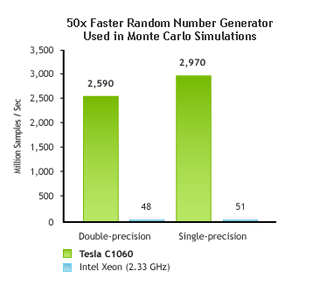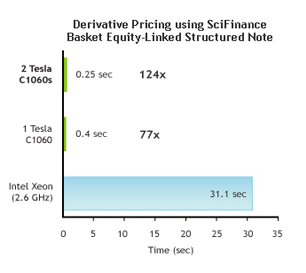# 計量財務針對GPU的NAG數值計算法 蒙地卡羅計價模型採用

 獨立軟體供應商(ISV) 說明 Murex 風險分析(MACS) MATLAB 數據平行數學 (MATLAB PCT, MDCS) Quantifi Solutions 資產投資組合風險 (Quantifi Risk), 信用風險 (Quantifi Counterparty Risk) Numerical Algorithms Group 亂數產生器 Wolfram Mathematica 符號運算分析(Mathematica) Streambase 複合事件處理 (StreamBase CEP Engine) Risk Management Solutions 巨災保險分析 Hanweck Associates 選擇權定價(Volera) SciComp, Inc 衍生性商品定價 (SciFinance) Xcelerit C++ 專用 GPU SDK，針對 Monte Carlo 和其他數值方法。

CUDA的計量財務軟體

CUDA技術報亦可參考

MATLAB is a registered trademark of The MathWorks, Inc.
Jacket is a trademark of AccelerEyes